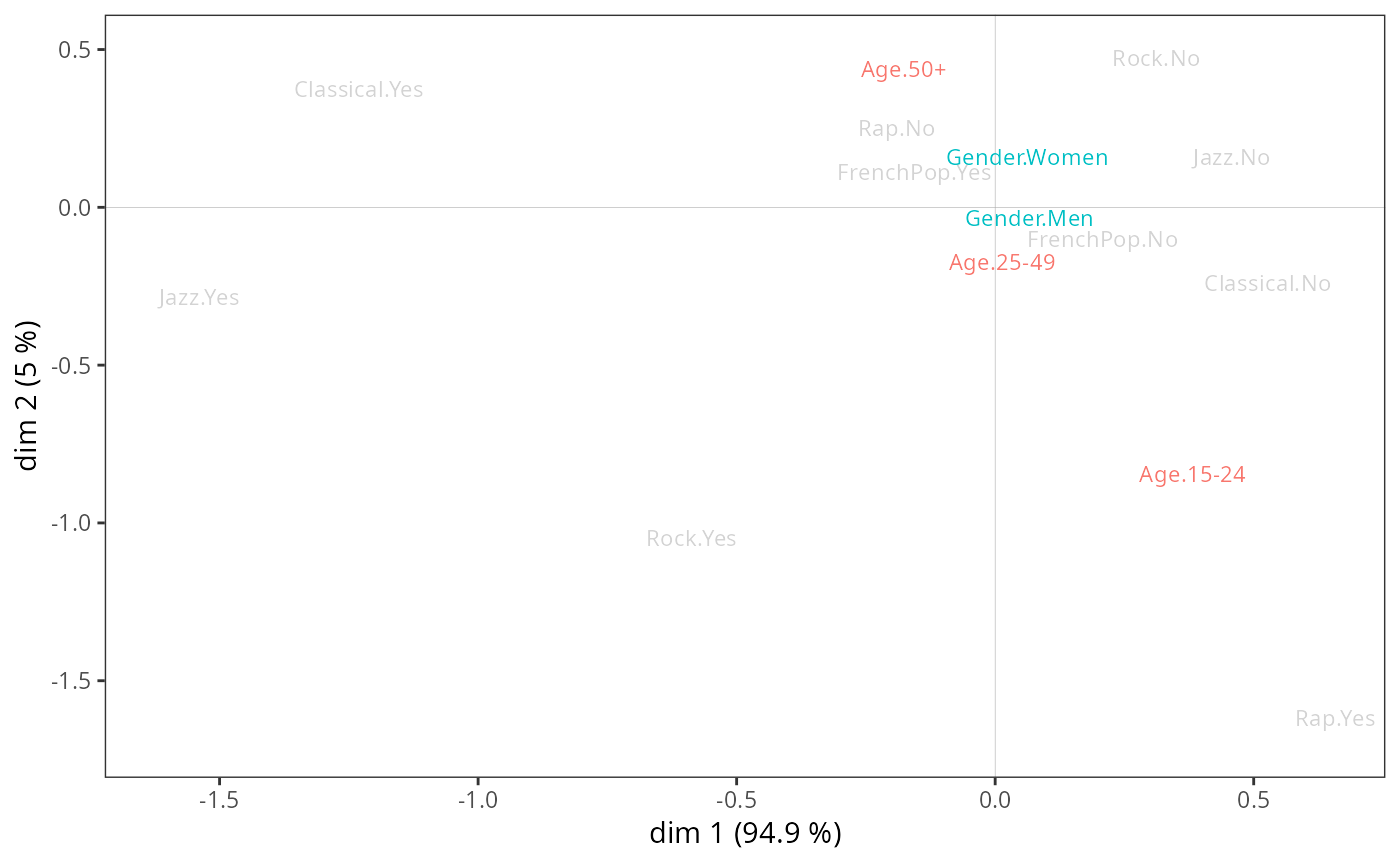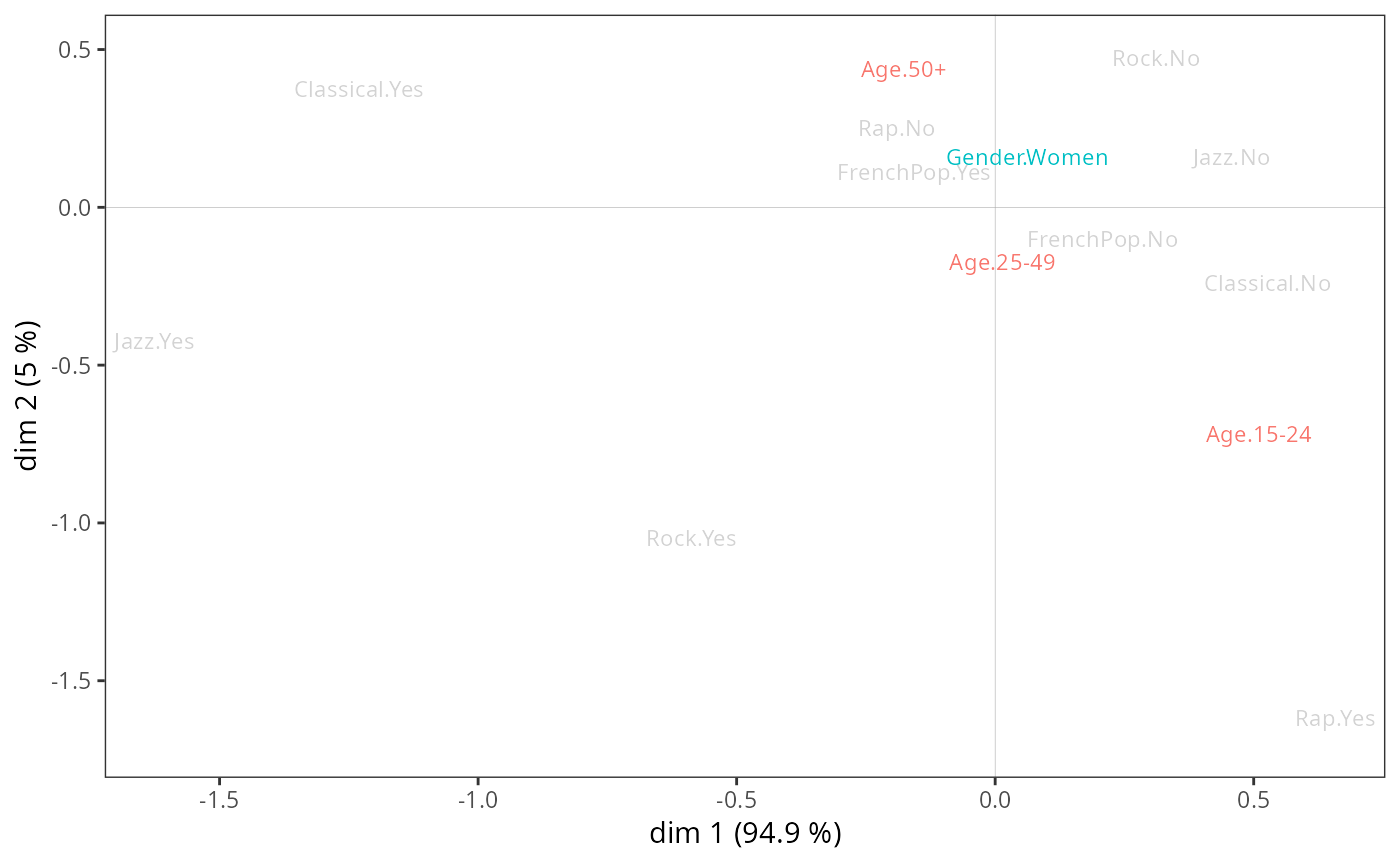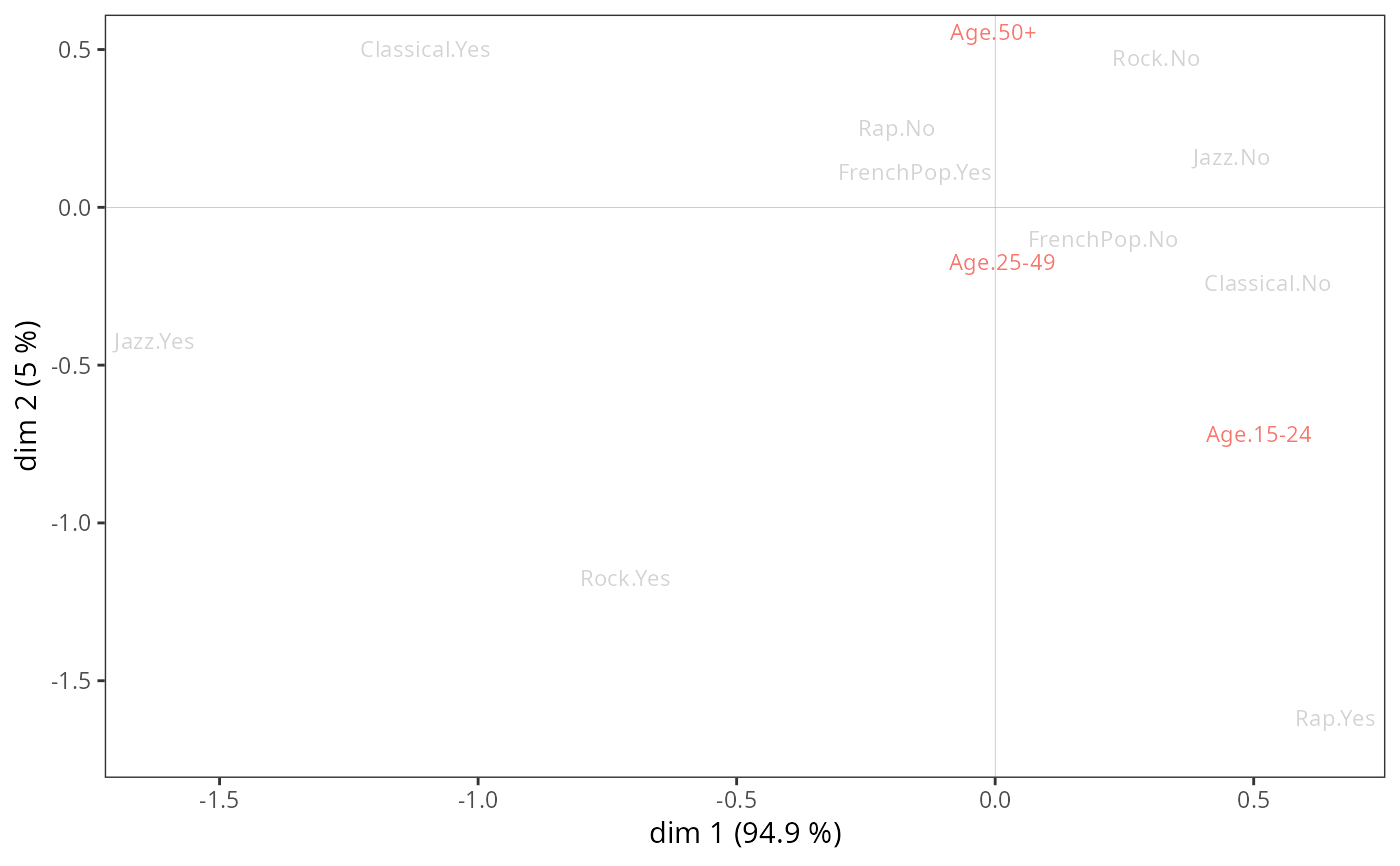Adds categorical supplementary variables to a MCA cloud of variables.

ggadd_supvars(p, resmca, vars, excl = NULL,
axes = c(1,2), col = NULL,
shapes = FALSE, prop = NULL, textsize = 3, shapesize = 6, vname = TRUE)

## Arguments

p

ggplot2 object with the cloud of variables

resmca

object of class MCA, speMCA, csMCA, stMCA or multiMCA.

vars

A data frame of categorical supplementary variables. All these variables should be factors.

excl

character vector of supplementary categories to exclude from the plot, specified in the form "namevariable.namecategory" (for instance "Gender.Men"). If NULL (default), all the supplementary categories are plotted.

axes

numeric vector of length 2, specifying the components (axes) to plot. Default is c(1,2).

col

character string. Color name for the labels (and the shapes if shapes=TRUE) of the categories. If NULL, the default palette of ggplot2 is used, with one color per variable.

shapes

Logical. If TRUE, symbols are used in addition to the labels of categories. Default is FALSE.

prop

If NULL, the size of the labels (if shapes=FALSE), or of the labels and the shapes (if shapes=TRUE) is constant. If 'n', the size is proportional the the weights of categories; if 'vtest1', the size is proportional to the test values of the categories on the first dimension of the plot; if 'vtest2', the size is proportional to the test values of the categories on the second dimension of the plot; if 'cos1', the size is proportional to the cosines of the categories on the first dimension of the plot; if 'cos2', the size is proportional to the cosines of the categories on the second dimension of the plot; if 'cos12', the size is proportional to the total cosines of the categories on the two dimensions of the plot.

textsize

Size of the labels of categories if shapes is TRUE, or if shapes is FALSE and prop is NULL. Default is 3.

shapesize

Size of the shapes if prop=NULL, maximum size of the shapes in other cases. Default is 6.

vname

Logical. If TRUE (default), the variable name is added as a prefix for the labels of the categories.

## Value

a ggplot2 object

## Note

Shapes and labels are colored according to the categories of the variable, using the default ggplot2 palette. The palette can be customized using any scale_color_* function, such as scale_color_brewer(), scale_color_grey() or scale_color_manual().

## Author

Nicolas Robette

ggcloud_variables, ggadd_supvar, ggadd_ellipses, ggadd_kellipses, ggadd_density, ggadd_interaction, ggsmoothed_supvar, ggadd_chulls, ggadd_corr

## Examples

# specific MCA of Music example data set
data(Music)
junk <- c("FrenchPop.NA", "Rap.NA", "Rock.NA", "Jazz.NA", "Classical.NA")
mca <- speMCA(Music[,1:5], excl = junk)cos <- supvars(mca, Music[, c("Gender","Age")])$cos2 drop <- rownames(cos)[cos$dim.1 < 0.001]